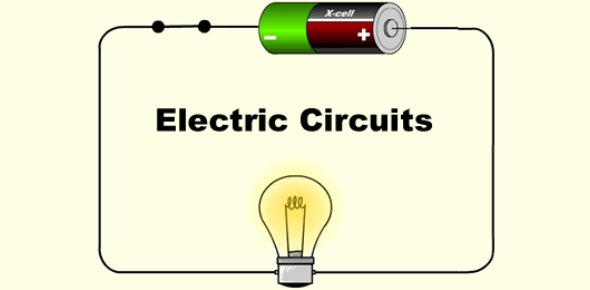# Electric Circuits Yr 9 Mod Hs Sci

55 Questions | Total Attempts: 2152SettingsAs part of the larger Science subject, electric circuits are taught. Electric energy is used in very many different ways, both directly and otherwise. The quiz below gauges your knowledge on electric circuits Yr. 9. All the best.

• 1.
Electric current in a/an ____________________ circuit travels in MORE THAN ONE path.
• A.

Series

• B.

Wire

• C.

Parallel

• D.

Connected

• 2.
In a/an ________________________ circuit, if one bulb burns out, the others will stay lit.
• A.

Parallel

• B.

Series

• 3.
In a _________________________ circuit, if one bulb burns out, the others will not work.
• A.

Parallel

• B.

Series

• 4.
A drawing that uses symbols to show how the parts of an electric circuit are connected is a/an _____________________________.
• A.

Nichrome wire

• B.

Electric diagram

• C.

Parallel circuit

• D.

Circuit diagram

• 5.
Which of the following is NOT a good insulator?
• A.

Plastic

• B.

Glass

• C.

Iron

• 6.
Which of the following is NOT a good conductor?
• A.

Iron

• B.

Copper

• C.

Plastic

• 7.
Electric current in a/an _____________________ circuit travels in ONE path.
• A.

Series

• B.

Parallel

• C.

Electric

• D.

Wire

• 8.
A material through which electric current CANNOT pass easily is a/an ____________________.
• A.

Tester

• B.

Insulator

• C.

Receptor

• D.

Conductor

• 9.
In order for a circuit to work, the path must be __________________.
• A.

Series

• B.

Paralled

• C.

Open

• D.

Closed

• 10.
What is current electricity?
• A.

Continuous flow of electrons in a circuit

• B.

A charge that is built up then dispersed

• C.

Energy stored within an item, that it then released

• 11.
Unlike  ___________________, current electricity needs an energy source and a complete path to follow.
• 12.
An _______ is a device for measuring resistance.
• 13.
What is measured by volts.
• A.

Current

• B.

Potential Difference

• C.

Resistance

• D.

Electricity

• E.

None of the above

• 14.
_____________ can flow when there is a complete circuit
• 15.
Materials through which electricity can pass are called ___________ conductors
• 16.
Which one is the odd one out
• A.

Glass

• B.

Tin

• C.

Copper

• D.

Silver

• 17.
The unit used for measuring electrical current is called a(an):
• A.

Electrode

• B.

Volt

• C.

Discharge

• D.

Ampere

• 18.
Lightning is caused by the build up of electrical charges in a cloud, also known as static electricity.
• A.

True

• B.

False

• 19.
The difference between DC and AC is that AC is
• A.

Bi-directional

• B.

Always constant

• C.

Safer

• D.

Faster

• 20.
What circuit symbol is this?
• A.

A Bulb

• B.

A Motor

• C.

A Fuse

• D.

A Cell

• 21.
Voltage does not split in a parallel circuit.
• A.

True

• B.

False

• 22.
In a Series circit, the current...?
• A.

Stays the same

• B.

Splits at a branch

• C.

Is given to the cell

• D.

Is shared amongst components

• 23.
A single battery is called a ----?
• 24.
Current is the amount of energy provided by the circuit, increasing this made the electrons travel faster.
• A.

True

• B.

False

• 25.
This is the symbol for:
• A.

An Ammeter

• B.

A Voltmeter

• C.

A Buzzer

• D.

An Electric Shock

Related TopicsBack to top